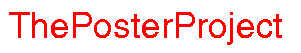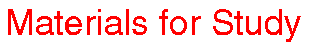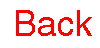Visualizing Women in Science, Mathematics and Engineering
• Home
• Posters
• Materials for Study
• Biographies
• The Design Team
•# What Is Scientific Truth?

What do you think of the following "theorems"?

1=2

#### Proof

Suppose a=b and neither is zero.

Then,

a²-b²=(a-b)(a+b)

Also,
a²-b²=a²-ab

So,
a²-ab=(a-b)(a+b)
a(a-b)=(a-b)(a+b)
a=a+b
a=a+a
a=2a
1=2

as required!

#### Theorem Two

Movement is impossible!

#### Proof

This is a proof by contradiction. I will assume that movement is possible and derive a contradiciton, thereby proving movement to be impossible.

So suppose movement is possible. In this case, the great Greek warrior Achilles can have a foot race with the very slow tortoise. Now, Achilles is the greatest warrior ever (imagine Rambo and the Terminator combined, and that dude wouldn't last ten seconds against Achilles. Achilles could flatten Goldberg with one arm tied behind his back. In fact, Achilles was so awesome that this Greek guy named Homer wrote a cool book about him called the Iliad.) It isn't really a fair race. I mean Achilles could smoke the tortoise who is slow and old and has to carry her house on her back. In the interests of fairness, we had better give the tortoise a little bit of a head start, let's say twenty feet.

Now we're ready to race. There's Achilles (the greatest warrior ever) and twenty feet ahead of him is the old tortoise. Bang! The starter's gun goes off and they're racing.

In a flash, Achilles covers the twenty feet separating him from the tortoise. But it takes him some positive amount of time to do it (albeit a very, very small amount of time) and in that little, tiny, miniscule amount of time the tortoise moves a little bit forward.

In a flash, Achilles covers that distance. But again, it takes him some small, tiny positive amount of time to do it. And, again, in that time the tortoise is able to move forward.

Never fear! Achilles is the greatest warrior ever. He could tear the arms off a gundark! In a flash, he covers that tiny distance ... but it takes him some tiny amount of time to do it ... and in that time the tortoise is able to move just the smallest amount forward.

But ...

... following this reasoning it is clear that Achilles can never overtake the poor, old, weak tortoise who must, therefore, win the race! This is clearly impossible, and so our starting assumption -- that movement was possible -- must be wrong.

Movement must therefore be impossible, which is what I was required to prove.

#### Theorem Three

There are exactly the same number of fractions as there are non-negative integers!

#### Proof

I will give one-to-one correspondences between fractions and non-negative integers, and this must show that there are exactly the same number of each!

Firstly, here is a one-to-one correspondence between non-negative integers and fractions. Let the non-negative integer n correspond to the fraction n/1. That is to say, 1 corresponds to 1/1, 2 corresponds to 2/1, 3 corresponds to 3/1, etc. This correspondence is clearly one-to-one: if a and b are non-negative integers corresponding to the same fraction then that means that a/1=b/1 so a=b.

Now all I have to do is give a one-to-one correspondence from fractions to non-negative integers. Here it is: let the fraction a/b correspond to the non-negative integer given by 2 raised to the power of a multiplied by 3 raised to the power of b. This correspondence is unique, because any given integer has only one factorization into prime powers (this is an elementary result from number theory. It means that given any number, there is only one way to write it as the product of powers of primes. 15=3 times 5, 18=2 times 3², etc.)

This means that fractions and non-negative integers must be in one-to-one correspondence, and hence there must be an equal number of each, which is what I said I would show.

Do you believe these "theorems"? If not, can you find the error in my reasoning? When you think you have the answers to these questions, check out my comments:

Hopefully, these three examples give you some idea of the dangers of relying solely on either intuition or formalism. In any "good" piece of math there is always a tension between intuition and the "details". It is very important to get both right.

Now that we're forewarned, we can consider what it means for something to be mathematically true .

In one sense, mathematical truth is a game. It is not meant to be profound. You start with a given set of axioms (axioms are statements which, for the purposes of the game, are assumed to be true) and a set of rules for manipulating these axioms. These rules are the rules of logic: suppose you know that A implies B and you also know that A is true, then there is a rule which says that B is true. To say that any given statement is true is to say that it is possible to form the statement by manipulating the axioms according to the rules of logic. It is not to say anything about the real world. This is the big difference between physics and math. Math is self-contained; it does not need to represent anything.

The axioms we use today are called the Zermelo-Frankel axioms. One of these axioms is called the Law of Excluded Middle, which states that if a given statement is not true, then its converse is. This is the axiom which allows us to use proof by contradiction (can you see why?) On one hand, this seems to be a perfectly sensible axiom (remember axioms are assumed to be true): if it is not true that Alice is taller than Zanele then it is true that Zanele is taller than Alice. But there are many examples from everyday life where the Law of Excluded Middle does not apply. Think of your own, then check out my suggestions.

It is important that a set of axioms be consistent. This means that it is not possible to derive both a statement and its converse from the axioms. For example, you do not want to be able to prove that there are infinitely many prime numbers AND that there are only finitely many prime numbers.

You may think this makes everything very black-and-white, or true-and-false so to speak. (Sorry, I couldn't resist!) But things are more subtle than that.

In the early years of the twentieth century, two English philosophers, Bertrand Russel and Alfred Whitehead, adopted the black-and-white view. They tried to formalize all of mathematics by carefully spelling out all axioms and then deducing every possible true statement, all of mathematics. During the course of this project, Russel came up with a famous paradox which bears his name.